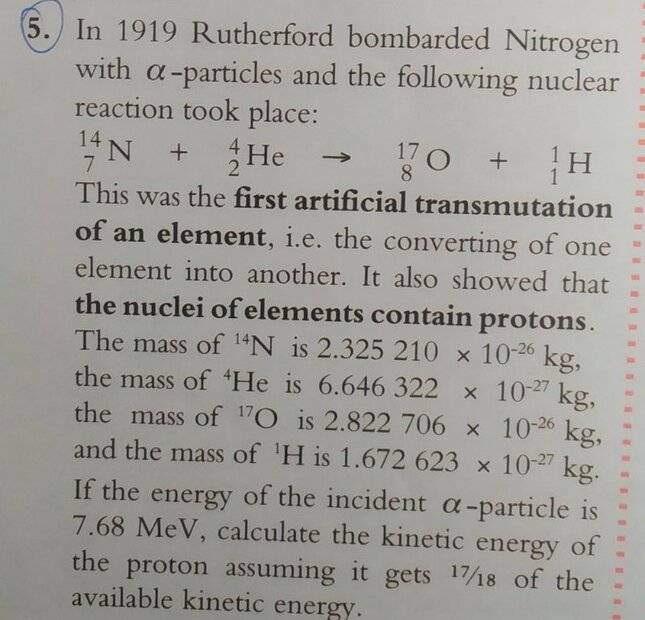# Mass-energy conservation in nuclear reactions

• voreryar

#### voreryar

Homework Statement
If the energy of the incident alpha-particle is 7.68 MeV, calculate the kinetic energy of the proton assuming it gets 17/18 of the available kinetic energy
Relevant Equations
E = mc^2I found the total mass of the reactants and the products, found the change in mass, used E=mc^2 and changed my answer from Joules to eV, but my answer is wrong. I'm guessing I have to do something with the energy of the alpha-particle given in the question

The answer is supposed to be: 6.58 MeV

Did you notice that the total mass of the products is greater than the total mass of the reactants? How do you account for this?

I found the total mass of the reactants and the products, found the change in mass, used E=mc^2 and changed my answer from Joules to eV, but my answer is wrong.
Hi @voreryar. Welcome to PF.

You need to show your working so we can spot any mistakes you have made. But it sounds like you haven’t applied conservation of mass-energy correctly.

I'm guessing I have to do something with the energy of the alpha-particle given in the question
Good guess!
Initial total rest mass ##=m_i##.
Final total rest mass ##= m_f##.
Initial total kinetic energy of all particles ##= K_i##.
Final total kinetic energy of all particles ##= K_f##.

What is the relationship between ##m_i, m_f, K_i## and ##K_f## in this problem?